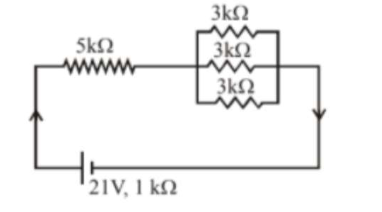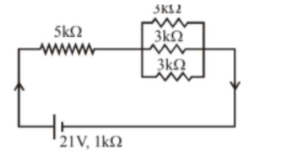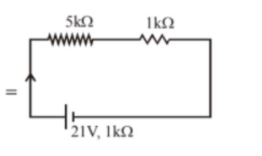# The value of $x$ to the nearest integer isQuestion:

The value of $x$ to the nearest integer is

In the figure given, the electric current flowing through the $5 \mathrm{k} \Omega$ resistor is 'x' mA.The value of $x$ to the nearest integer is

Solution:

(3)$I=\frac{21}{5+1+1}=3 \mathrm{~mA}$